# Carleman theorem

Carleman's theorem on quasi-analytic classes of functions is a necessary and sufficient condition for quasi-analyticity in the sense of Hadamard, discovered by T. Carleman

(see also ). A classof real-valued infinitely differentiable functionson an interval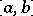is said to be quasi-analytic in the sense of Hadamard if the equalities,at some fixed point,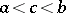, imply that. The statement of the theorem: The classis quasi-analytic if and only if(1)

where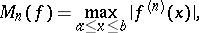is a constant, and the sequence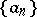satisfies one of the equivalent conditions: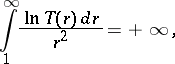(2)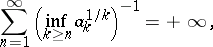where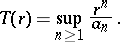This is one of the first definitive results in the theory of quasi-analytic classes of functions. Quasi-analytic classes defined by (1), (2) are often called Carleman classes.

Carleman's theorem on conditions of well-definedness of moment problems: If the sequence of positive numbers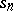,satisfies the conditionthen the moment problem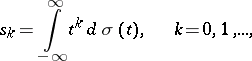(3)

is well-defined. This means that there exists a non-decreasing function,, satisfying the equations (3), which is unique up to addition by any function which is constant in a neighbourhood of each point of continuity of it. This theorem was established by T. Carleman (see , ).

Carleman's theorem on uniform approximation by entire functions: If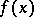is any continuous function on the real line and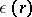,, is a positive continuous function decreasing arbitrarily rapidly as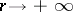, then there exists an entire function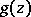of the complex variablesuch thatThis theorem, established by T. Carleman , was the starting point in the investigations into approximation by entire functions. In particular, a continuumin the-plane is said to be a Carleman continuum if for any continuous complex functiononand an arbitrary rapidly decreasing positive function(as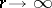) with a positive infimum on any finite interval, there exists an entire functionsuch that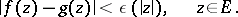Necessary and sufficient conditions for a closed set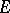to be a Carleman continuum were obtained in a theorem by M.V. Keldysh and M.A. Lavrent'ev (see ). An example of a Carleman continuum is a closed set consisting of rays of the form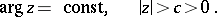Carleman's theorem on the approximation of analytic functions by polynomials in the mean over the area of a domain: Letbe a finite domain in the complex-plane,, bounded by a Jordan curve, and let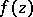be a regular analytic function insuch thatThen there exists for anya polynomial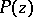such that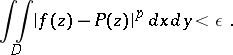This result was established by T. Carleman . Similar results also hold for approximation with an arbitrary positive continuous weight, in which case the boundarycan be of a more general nature. The system of monomials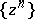,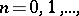is complete with respect to any such weight. Orthogonalization and normalization of this system gives polynomialsof degree, which are often called Carleman polynomials.